top of page## The Power of Multiplication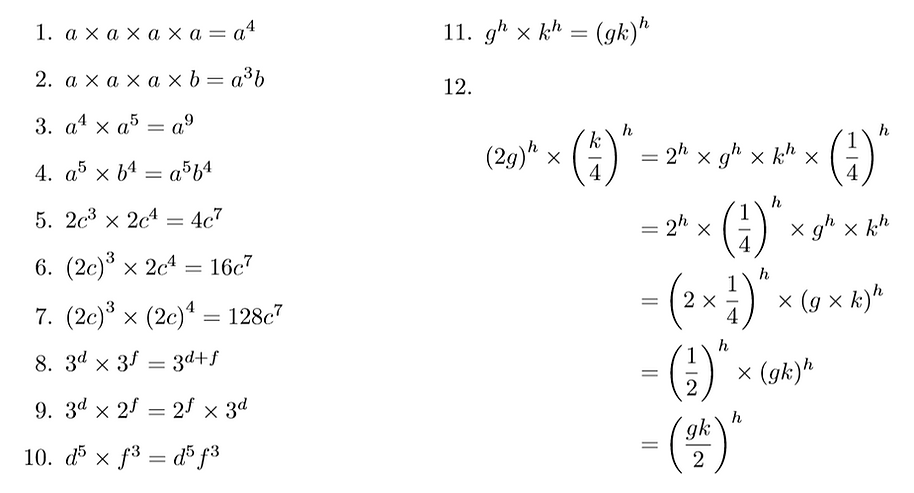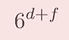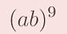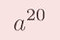As well as the correct solutions, common incorrect responses are given here. Make sure you understand the misconception at work in each of these mistakes and consider how you could explain them away so that you are not fooled by these tempting mistakes in future.

## The Power of Division

As well as the correct solutions, common incorrect responses are given here. Make sure you understand the misconception at work in each of these mistakes and consider how you could explain them away so that you are not fooled by these tempting mistakes in future. Also included are some notes on the consequent behaviour of indices that becomes apparent as we investigate division.Just by following this division rule, we can see there are consequences for the power of 0 and negative powers. We shall investigate these next.

## The Power of Negatives

As we learn more shortcuts in the world of indices, we find there are more and more ways to reason through the evaluation of different expressions. Therefore, some of these solutions are given in more than one way, usually once using actual division and once using only the laws of indices already covered. Take some time to make sure you understand both points of view as well as how and why they are infact the same. Notice that Q11 here is another look at Q10 from above which we can now express in a more pleasing manner now that we know more about negatives in powers.Notice that Q9 here demonstrates that the product of reciprocals is the same as the reciprocal of the product. Similarly, Q10 shows that the quotient of reciprocals is the same as the reciprocal of the quotient.

## Power to a Power## The Power of Fractions

Q3: Note that thinking this way around is far simpler than trying to cube root the square of 27, but since we know this would have the same value we can deduce that:Q4/5: Note that we search for square factors to simplify surds involving square roots and cube factors to simplify surds involving cube roots.

Q6: Again note that thinking this way around is far, far simpler than trying to fifth root the eighth power of 32 (a v large number), but we know this would have the same value and we can otherwise skirt around the problem using our laws of indices: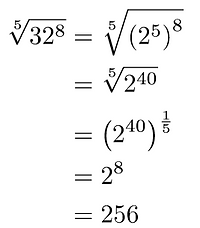Q11: The trick to this is spotting that the base to go for is the square root of 10 as opposed to just 10. It is extremely useful to remember that anything which can be expressed as a power of 10 can also be expressed as a power of any root of 10. Obviously this is not unique to the number 10 but it is a universal property of all integers?## The Power to Puzzle

There are lots of ways to solve these puzzles so those given here are only a suggestion of some valid reasoning. There are more efficient ways to solve some of these problems using mathematics from the A level syllabus, however the solutions provided use GCSE content since this site is for those fluent in GCSE but still preparing for A level maths. We are always interested to see alternative, clever & elegant solutions to our problems so please send yours to us @MissCowleyMaths and you may well feature here in future.bottom of page